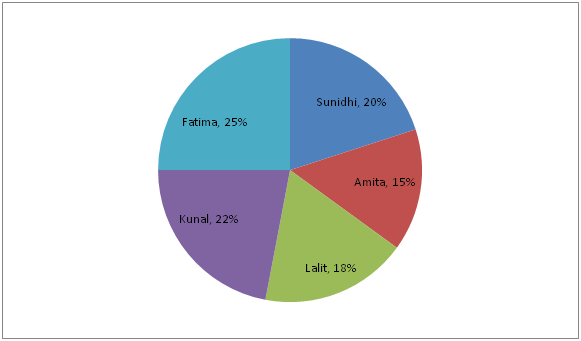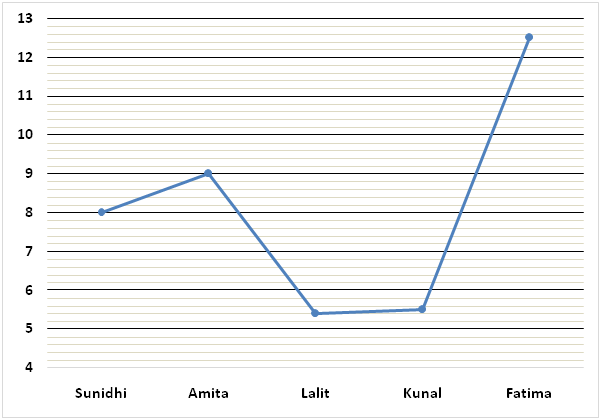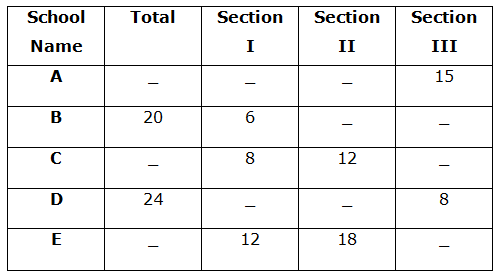# SBI PO Quantitative Aptitude Questions 2019 (Day-23) High Level New Pattern

SBI PO 2019 Notification is about to come and it is the most awaited exam among the aspirants. We all know that new pattern questions are introducing every year in the SBI PO exam. Further, the questions are getting tougher and beyond the level of the candidate’s expectations.

Our IBPS Guide is providing High-Level New Pattern Quantitative Aptitude Questions for SBI PO 2019 so the aspirants can practice it on a daily basis. These questions are framed by our skilled experts after understanding your needs thoroughly. Aspirants can practice these high-level questions daily to familiarize with the exact exam pattern. We wish that your rigorous preparation leads you to a successful target of becoming SBI PO.

[WpProQuiz 5263]

### Click Here for SBI PO Pre 2019 High-Quality Mocks Exactly on SBI Standard

Directions (1 – 5): Study the following information carefully and answer the questions given below:

The following pie chart represents percentage wise distribution of the part of the work completed by five students.The following line graph represents number of days taken by them to complete their part of the work.1) Lalit, Kunal and Fatima started the work. Lalit left five days before the work completed and Kunal left ten days before the work completed and Fatima worked till the work completed. Find the total number of days?

a) 229/7 days

b) 231/9 days

c) 235/14 days

d) 238/5 days

e) None of these

2) Sunidhi started the work. Amita and Lalit accompanies her on alternate days starting with Amita i.e. on first day Sunidhi and Amita works, on second day Sunidhi and Lalit works and so on. In how many days the work will be completed?

a) 20 days

b) 18 days

c) 12 days

d) 16 days

e) None of these

3) Efficiency of Sarita is 25% more than the efficiency of Kunal. Find the number of days taken by Sarita, Amita and Fatima together to complete the work.

a) 70/12 days

b) 150/13 days

c) 90/7 days

d) 113/5 days

e) None of these

4) Sunidhi and Rakesh started the work and left after 20 days. Find the number of days taken by Nisha to complete remaining part of the work.

Statement I: Rakesh and Nisha together can complete one third of the work in 300/29 days.

Statement II: Risabh alone can complete the work in 35 days. With the help of Nisha, he can complete the work in 315/16 days.

a) Statement I alone is sufficient to answer the question, but the statement II alone is not sufficient.

b) Statement II alone is sufficient to answer the question, but the statement I alone is not sufficient.

c) Either statement I alone or statement II alone is sufficient to answer the question.

d) Both statements I and II together are needed to answer the question.

e) Both statements I and II together are not sufficient to answer the question.

5) Efficiency of Raghav and Kunal together is what percent of the efficiency of Meena and Fatima together?

Statement I: Kunal and Meena together can complete the work in 300/17 days. With the help of Raghav, they can complete the work in 100/9 days.

Statement II: Efficiency of Raghav is twice the efficiency of Meena.

a) Statement I alone is sufficient to answer the question, but the statement II alone is not sufficient.

b) Statement II alone is sufficient to answer the question, but the statement I alone is not sufficient.

c) Either statement I alone or statement II alone is sufficient to answer the question.

d) Both statements I and II together are needed to answer the question.

e) Both statements I and II together are not sufficient to answer the question.

Directions (6 – 10): Study the following graph carefully and answer the given questions.

The table shows the number of students participated in different sections from different schools.6) A group of four students has to form from school C such that the group contains one student in each section and the remaining from any of the section. The number of ways in which this can be possible is 25920. Find the number of students in section III from school C?

a) 15

b) 12

c) 10

d) 20

e) 18

7) A group of two students has to form from school E such that the group contains at least one student in section III. The number of ways in which this can be possible is 195. A committee of five students is to be formed from school E such that the committee contains 2 students from section I, 1 student from section II and 2 students from section III. Find the number of ways in which this can be possible?

a) 15920

b) 16478

c) 23420

d) 17820

e) None of these

8) Find the total number of students in school A?

Statement I: All the students in section II from school A is handshaking with each other and the total number of handshakes is 105.

Statement II: All the students in school A is handshaking with each other and the total number of handshakes is 780.

a) Only I

b) Only II

c) Both I and II

d) Either I or II

e) Neither I nor II

9) In school B, number of students in section III is 75% of the number of students in section II.

Quantity I: In committee P, five students is to be formed from school B such that the committee contains 1 student from each section and the remaining students from any of the section.

Quantity II: In committee Q, five students is to be formed from school B such that committee contains at least one student from section I.

a) Quantity I > Quantity II

b) Quantity I < Quantity II

c) Quantity I ≥ Quantity II

d) Quantity I ≤ Quantity II

e) Quantity I = Quantity II (or) Relationship cannot be determined

10) A committee of three members is to be formed from each school B and school D. Number of possible ways of a committee contains three students in section III from school D is what percentage of the number of possible ways of committee contains three students in section I from school B?

a) 180%

b) 380%

c) 160%

d) 280%

e) None of these

Direction (1-5) :

Lalit can do 18% of the work in 5.4 days

=>Lalit can do 1% of the work in 5.4/18 days

=>Lalit can do 100% of the work in 5.4/18 x 100 = 30 days.

Kunal can do 22% of the work in 5.5 days

=>Kunal can do 1% of the work in 5.5/22 days

=>Kunal can do 100% of the work in 5.5/22 x 100 = 25 days.

Fatima can do 25% of the work in 12.5 days

=> Fatima can do 1% of the work in 12.5/25 days

=> Fatima can do 100% of the work in 12.5/25 x 100 = 50 days.

Let, the work completed in n days

(n – 5)/30 + (n – 10)/25 + n/50 = 1

=> (5n – 25 + 6n – 60 + 3n)/150 = 1

=> 14n – 85 = 150

=> 14n = 150 + 85

=> 14n = 235

=> n = 235/14 days

Sunidhi can do 20% of the work in 8 days

=>Sunidhi can do 1% of the work in 8/20 days

=>Sunidhi can do 100% of the work in 8/20 x 100 = 40 days.

Amita can do 15% of the work in 9 days

=>Amita can do 1% of the work in 9/15 days

=>Amita can do 100% of the work in 9/15 x 100 = 60 days.

Lalit can do 18% of the work in 5.4 days

=>Lalit can do 1% of the work in 5.4/18 days

=>Lalit can do 100% of the work in 5.4/18 x 100 = 30 days.

Part of the work completed in 2 days = 1/40 + 1/60 + 1/40 + 1/30

= (3 + 2 + 3 + 4)/120

= 12/120

= 1/10

Time taken to complete 1/10 work = 2 days

=> Time taken to complete 1 work = 2 x 10 = 20 days

Kunal can do 22% of the work in 5.5 days

=>Kunal can do 1% of the work in 5.5/22 days

=>Kunal can do 100% of the work in 5.5/22 x 100 = 25 days.

1/Sarita = 1/25 x 125/100

=> 1/Sarita = 1/20

Amita can do 15% of the work in 9 days

=>Amita can do 1% of the work in 9/15 days

=>Amita can do 100% of the work in 9/15 x 100 = 60 days.

Fatima can do 25% of the work in 12.5 days

=> Fatima can do 1% of the work in 12.5/25 days

=> Fatima can do 100% of the work in 12.5/25 x 100 = 50 days.

Let, required number of days = n

n x (1/20 + 1/60 + 1/50) = 1

=>n x (15 + 5 + 6)/300 = 1

=> n = 300/26

=> n = 150/13 days

Sunidhi can do 20% of the work in 8 days

=>Sunidhi can do 1% of the work in 8/20 days

=>Sunidhi can do 100% of the work in 8/20 x 100 = 40 days.

From I:

300/29 x (1/Rakesh + 1/Nisha) = 1/3

=> 1/Rakesh + 1/Nisha = 1/3 x 29/300

=> 1/Rakesh + 1/Nisha = 29/900

From II:

1/Risabh = 1/35

1/Risabh + 1/Nisha = 16/315

=> 1/35 + 1/Nisha = 16/315

=>1/Nisha = 16/315 – 1/35

=> 1/Nisha = (16 – 9)/315

=> 1/Nisha = 7/315

=> 1/Nisha = 1/45

From I and II:

1/Rakesh + 1/45 = 29/900

=> 1/Rakesh = 29/900 – 1/45

=> 1/Rakesh = (29 – 20)/900

=> 1/Rakesh = 9/900

=> 1/Rakesh = 1/100

Let, required number of days = n

20/40 + 20/100 + n/45 = 1

=> ½ + 1/5 + n/45 = 1

=> n/45 = 1 – ½ – 1/5

=> n/45 = (10 – 5 – 2)/10

=> n = 45 x 3/10

=> n = 27/2 days

Hence, both statements I and II together are needed to answer the question.

From I:

Kunal can do 22% of the work in 5.5 days

=>Kunal can do 1% of the work in 5.5/22 days

=>Kunal can do 100% of the work in 5.5/22 x 100 = 25 days.

1/Kunal + 1/Meena = 17/300

=> 1/25 + 1/Meena = 17/300

=> 1/Meena = 17/300 – 1/25

=> 1/Meena = (17 – 12)/300

=> 1/Meena = 5/300

=> 1/Meena = 1/60

1/Kunal + 1/Meena + 1/Raghav = 9/100

=> 1/25 + 1/60 + 1/Raghav = 9/100

=> 1/Raghav = 9/100 – 1/25 – 1/60

=> 1/Raghav = (27 – 12 – 5)/300

=> 1/Raghav = 10/300

=> 1/Raghav = 1/30

Required percentage = [(1/30 + 1/25) / (1/60 + 1/50)] x 100

= [(10 + 12)/300]/[(5 + 6)/300] x 100

= 22/11 x 100

= 200%

Statement II:

1/Raghav = 2/Meena

Hence, statement I alone is sufficient to answer the question, but the statement II alone is not sufficient.

Direction (6-10) :

No of students in section III from school C = x

Total number of students in school C = x + 8 + 12 = 20 + x

Number of possible ways = 8C1 * 12C1 * xC1 * (20 + x – 3) C1 = 25920

8 * 12 * x * (17+x) = 25920

17x + x2 = 270

= > x2 + 17x – 270 = 0

= > x2 – 10x + 27x – 270 = 0

= > x (x – 10) + 27 (x – 10) = 0

= > x = 10, – 27 (Eliminate the –Ve value)

Number of students in section III from school C = 10

Number of students in section III from school E = y

Total number of students in school E = (y + 12 + 18)

= y + 30

Number of possible ways = yC1 * 30C1 + yC2 = 195

= > y * 30 + (y*(y-1)/(1 *2)) = 195

= > 30y + (y2 – y)/2 = 195

= > 60y + y2 – y = 390

= > y2 + 59y – 390 = 0

= > y2 + 65y – 6y – 390 = 0

= > y (y + 65) – 6 (y + 65) = 0

= > (y + 65) (y – 6) = 0

= > y = 6, -65 (Eliminate –Ve value)

Total number of students in school E = 30 + 6 = 36

Required number of ways = 12C2 * 18C1 * 6C2

= (12*11/1*2) *18 *(6*5/1*2)

= 6*11*18*3*5

= 17820

From I,

Number of students in section II from school A = n

Number of handshakes = nC2 = 105

= > [n*(n -1)] / (1 * 2) = 105

= > n2 – n = 210

= > n2 – n – 210 = 0

= > n2 – 15n + 14n – 210 = 0

= > n (n – 15) + 14 (n – 15) = 0

= > (n – 15) + (n – 14) = 0

= > n = 15, -14 (Eliminate –Ve value)

From statement I, We have to find only number of students in section II from school A. We can’t find the total number of students.

Hence, statement I alone is not sufficient to answer the given question.

From II,

Total number of students in school A = x

Number of handshakes = xC2 = 780

= > x * (x – 1)/1*2 = 780

= > x2 – x = 780*2

= > x2 – x – 1560 = 0

= > x2 – 40x + 39x – 1560 = 0

= > x (x – 40) + 39 (x – 40) = 0

= > (x – 40) (x + 39) = 0

= > x = 40, -39 (Eliminate –Ve value)

Total number of students in school A is 40.

Hence, Statement II alone is sufficient to answer the given question.

Number of students in section II from school B = x

Number of students in section III from school B = 75/100 * x

= 3x/4

Total number of students in section II and III from school B = 20 – 6 = 14

= > x + 3x/4 = 14

= > 7x/4 = 14

= > x = 8

Number of students in section III from school B = 8 * 75/100 = 6

Quantity I:

Number of ways = 6C1*8C1*6C1*(20-3)C2

= 6 * 8 * 6 *(17*16/1*2)

= 6*8*6*17*8

= 39168

Quantity II: In committee Q, five students is to be formed from school B such that committee contains at least one student from section I.

Number of ways = 6C1*14C4 + 6C2*14C3 + 6C3*14C2 + 6C4*14C1 + 6C5

= 6006 + 5460 + 1820 + 210 + 6

= 13502

Hence, Quantity I > Quantity II

Number of ways in school D = 8C3

= 8*7*6/1*2*3

= 56

Number of ways in school B = 6C3

= 6*5*4/1*2*3

= 20

Required percentage = 56/20 * 100 = 280%

### Click Here for SBI PO Pre 2019 High-Quality Mocks Exactly on SBI Standard